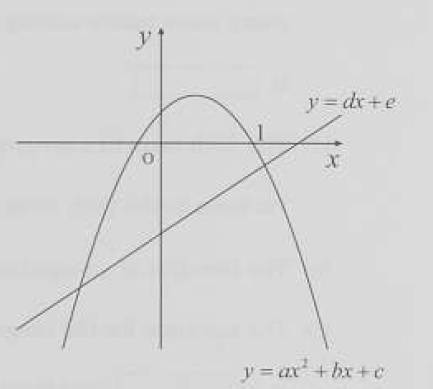# Second degree functions

• B
help
TL;DR Summary
judge whether the following expressions are larger than, smaller than or equal to zero
judge whether the following expressions are larger than, smaller than or equal to zero
i) a+b+c
ii)a-b+c
iii)4a+2b+c
iv)c-e

I know that <0, b> 0, c> 0, d> 0 and e <0 but I don't know what to doHomework Helper
Gold Member
Summary:: judge whether the following expressions are larger than, smaller than or equal to zero

I know that <0, b> 0, c> 0, d> 0 and e <0 but I don't know what to do
inconclusive on most of those.

Mentor
2022 Award
Summary:: judge whether the following expressions are larger than, smaller than or equal to zero

judge whether the following expressions are larger than, smaller than or equal to zero
i) a+b+c
ii)a-b+c
iii)4a+2b+c
iv)c-e

I know that <0, b> 0, c> 0, d> 0 and e <0 but I don't know what to do

View attachment 263235
Note that e.g. ##a+b+c=y(1)## or ##c=y(0)##. Then the equation ##y(x)=0## has two solutions. Which are the solutions in general, and what does it mean that there are two real solutions?

•help
help
thanks a lot!

Mentor
inconclusive on most of those.
Which one is inconclusive? All of them can be taken from the graph.

Homework Helper
Gold Member
I was only going by this:
Summary:: judge whether the following expressions are larger than, smaller than or equal to zero

whether the following expressions are larger than, smaller than or equal to zero
i) a+b+c
ii)a-b+c
iii)4a+2b+c
iv)c-e

I know that a<0, b> 0, c> 0, d> 0 and e <0
The "know" part only tells if a number is POSITIVE or NEGATIVE.

Looking at (i),
a+b+c
neg + pos + pos
we do not know how the neg compares to the two pos values.

I see no reason why the graph was shown. It appears to be separate from the given description of O.P. I did not make use of it, so this is my possible misunderstanding.

Mentor